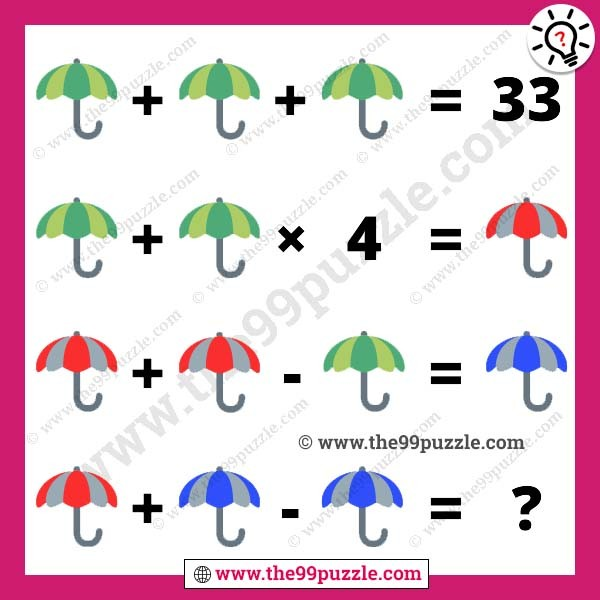# Umbrella picture math equation with answer – Puzz220

Umbrella picture math equation for intelligence. Can you solve this brain teaser math picture puzzle? Logical and tricky picture puzzles are interesting for students. Math picture puzzles can test your visual and mathematical skills. When you are under more work pressure, this type of puzzle can relax your mind. Always puzzle-solving helps you smarter and genius. These puzzles are for all-level students. Many times you fail to solve these easy puzzles. These puzzles are viral on social media. In this picture, you see three different color umbrellas. You have to find out each value and solve the last picture equation.###### Explanation:

Green Umbrella + Green Umbrella + Green Umbrella = 33

Green Umbrella + Green Umbrella × 4 = Red Umbrella

Red Umbrella + Red Umbrella – Green Umbrella = Blue Umbrella

Red Umbrella + Blue Umbrella – Blue Umbrella = ?

Green Umbrella = 11

Red Umbrella = 55

Blue Umbrella = 99

11 + 11 + 11 = 33

11 + 11 × 4 = 55

55 + 55 – 11 = 99

55 + 99 – 99 = 55